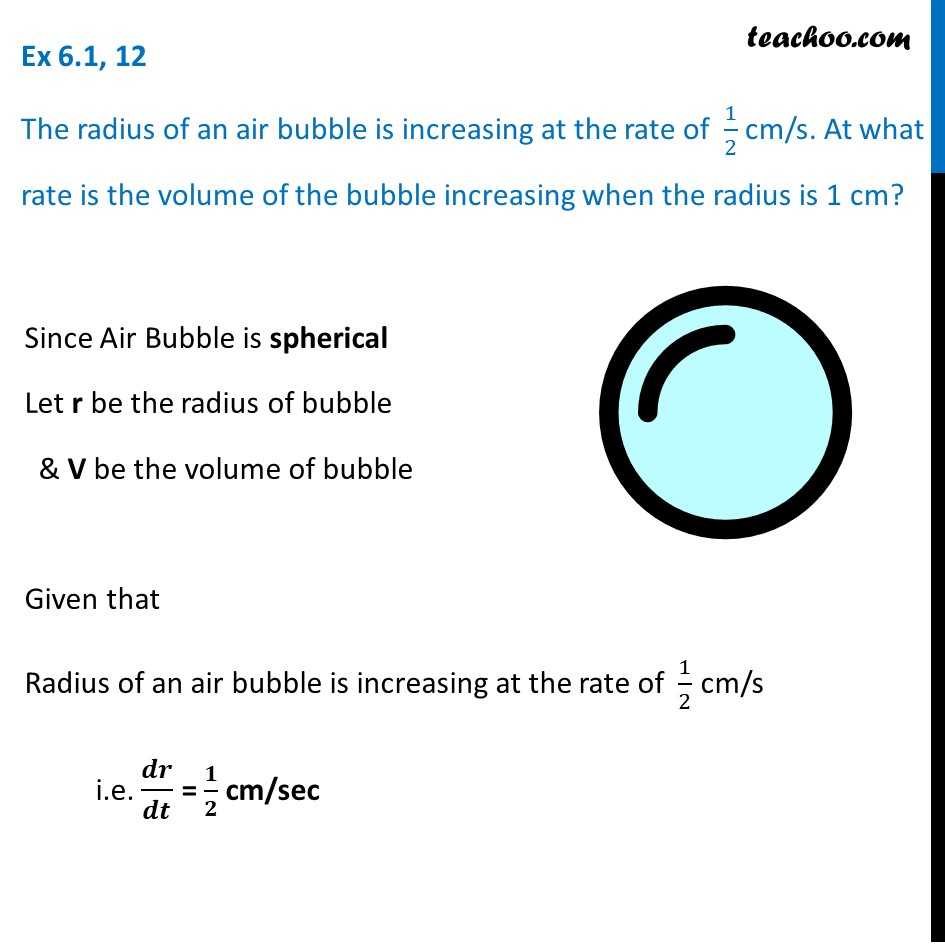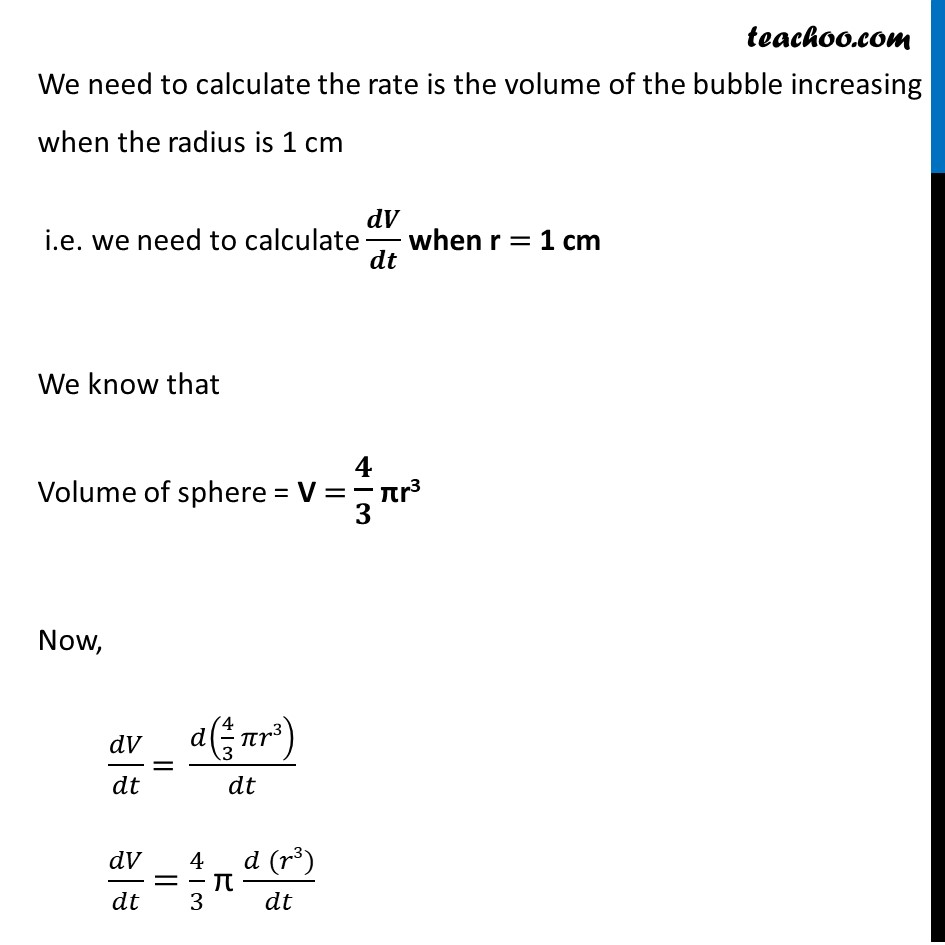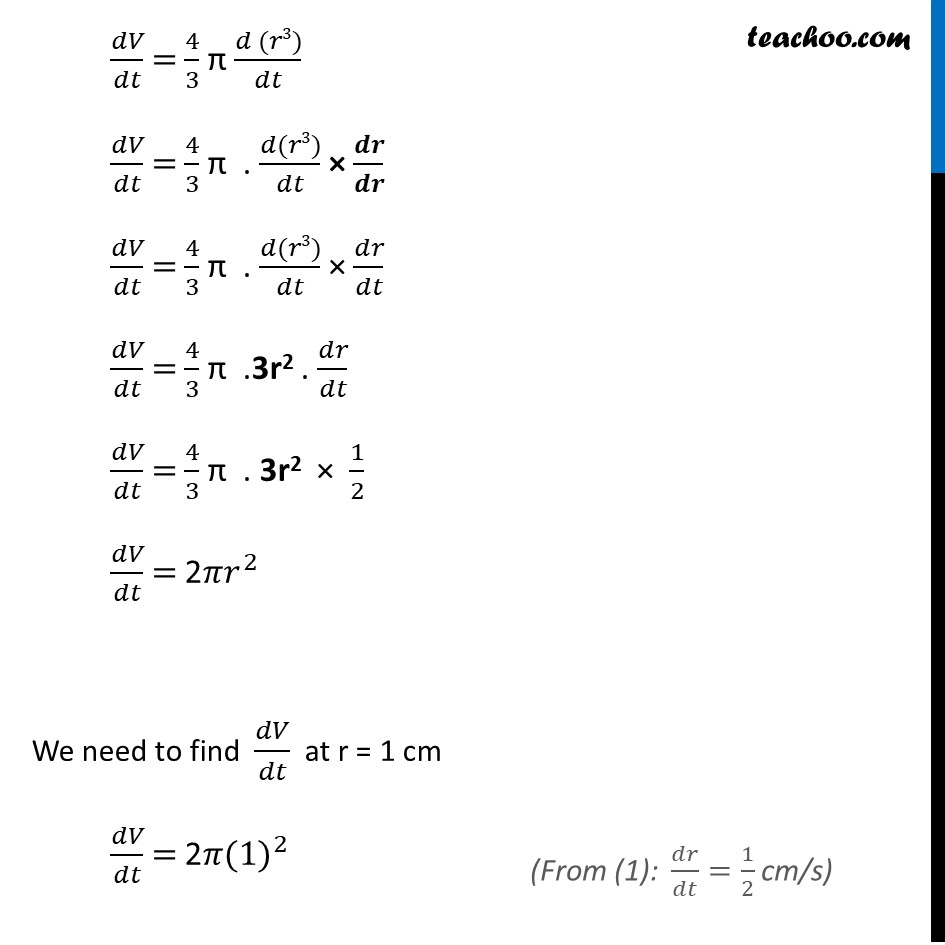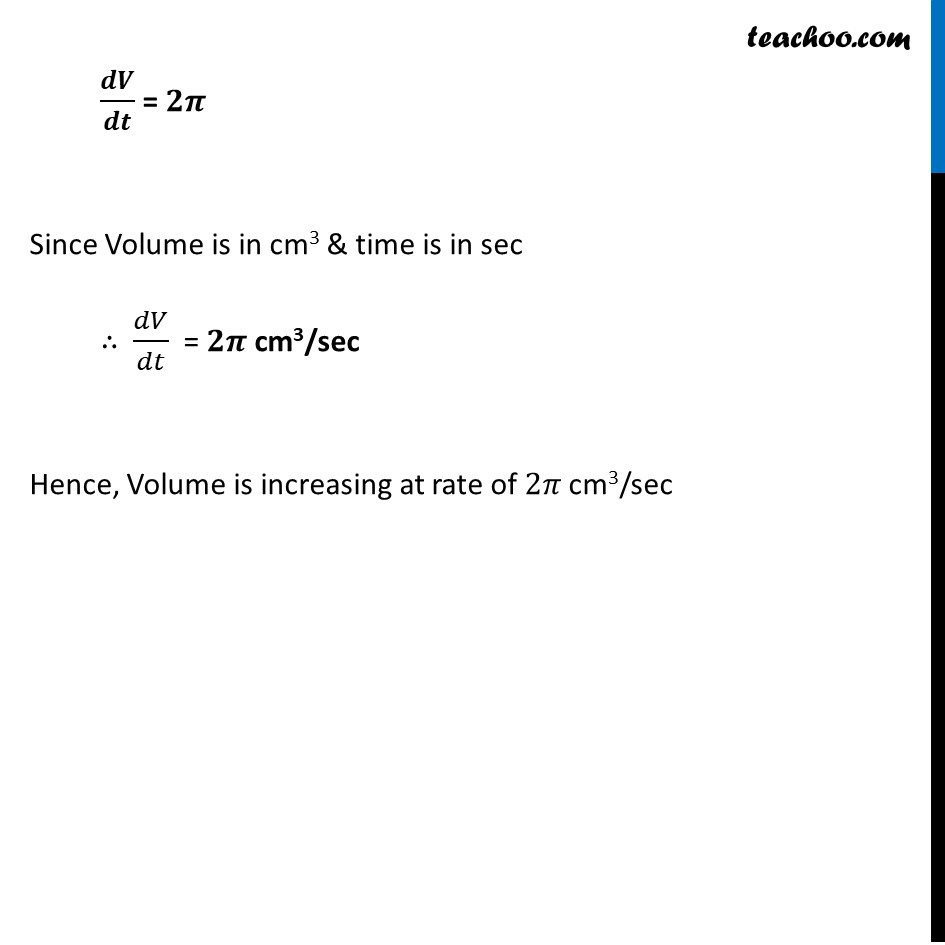Finding rate of change

Chapter 6 Class 12 Application of Derivatives
Concept wiseLearn in your speed, with individual attention - Teachoo Maths 1-on-1 Class

### Transcript

Ex 6.1, 12 The radius of an air bubble is increasing at the rate of 1/2 cm/s. At what rate is the volume of the bubble increasing when the radius is 1 cm?Since Air Bubble is spherical Let r be the radius of bubble & V be the volume of bubble Given that Radius of an air bubble is increasing at the rate of 1/2 cm/s i.e. 𝒅𝒓/𝒅𝒕 = 𝟏/𝟐 cm/sec We need to calculate the rate is the volume of the bubble increasing when the radius is 1 cm i.e. we need to calculate 𝒅𝑽/𝒅𝒕 when r = 1 cm We know that Volume of sphere = V = 𝟒/𝟑 πr3 Now, 𝑑𝑉/𝑑𝑡 = 𝑑(4/3 𝜋𝑟3)/𝑑𝑡 𝑑𝑉/𝑑𝑡 = 4/3 π (𝑑 (𝑟3))/𝑑𝑡 𝑑𝑉/𝑑𝑡 = 4/3 π (𝑑 (𝑟3))/𝑑𝑡 𝑑𝑉/𝑑𝑡 = 4/3 π . (𝑑(𝑟3))/𝑑𝑡 × 𝒅𝒓/𝒅𝒓 𝑑𝑉/𝑑𝑡 = 4/3 π . (𝑑(𝑟3))/𝑑𝑡 × 𝑑𝑟/𝑑𝑡 𝑑𝑉/𝑑𝑡 = 4/3 π .3r2 . 𝑑𝑟/𝑑𝑡 𝑑𝑉/𝑑𝑡 = 4/3 π . 3r2 × 1/2 𝑑𝑉/𝑑𝑡 = 2𝜋𝑟^2 We need to find 𝑑𝑉/𝑑𝑡 at r = 1 cm 𝑑𝑉/𝑑𝑡 = 2𝜋〖(1)〗^2 ("From (1): " 𝑑𝑟/𝑑𝑡=1/2 cm/s) 𝒅𝑽/𝒅𝒕 = 𝟐𝝅 Since Volume is in cm3 & time is in sec ∴ 𝑑𝑉/𝑑𝑡 = 𝟐𝝅 cm3/sec Hence, Volume is increasing at rate of 2𝜋 cm3/sec